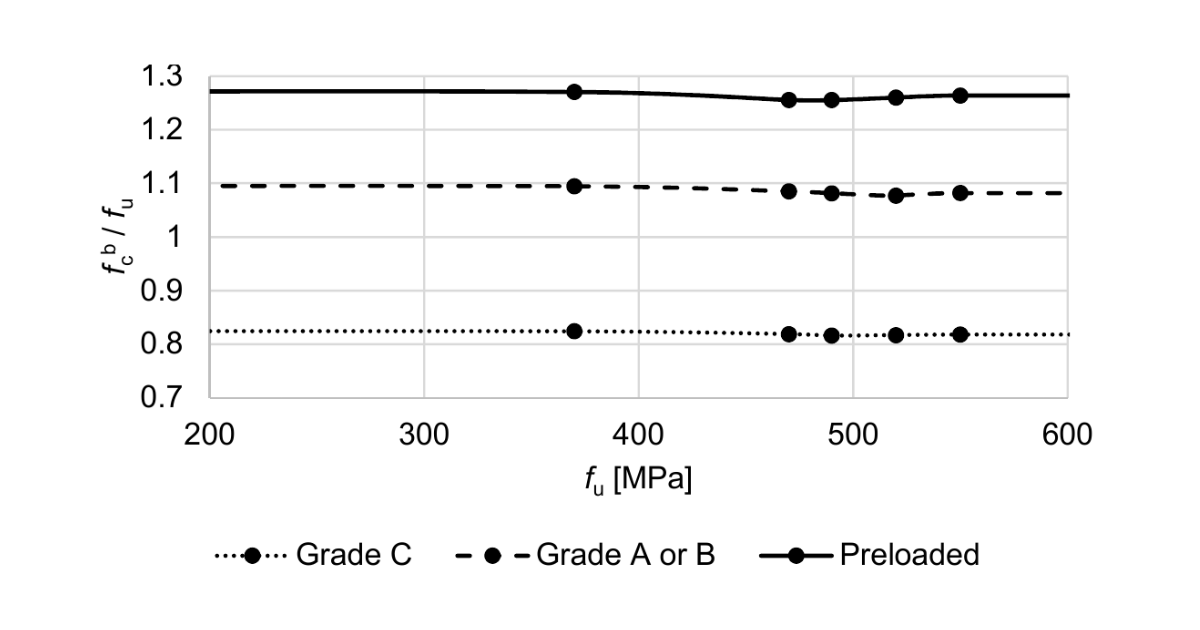### Choose language# Code-check of bolts and preloaded bolts according to Hong Kong Code

$$Shear capacityShear capacity of preloaded bolts is determined according to Cl. 9.3.6.2 as:$P_{SL} = 0.9 \cdot K_s \cdot \mu \cdot P_0$ where: $$K_s$$ – hole coefficient taken as for standard holes $$K_s = 1.0$$ for oversized holes $$K_s = 0.85$$ for slotted holes $$K_s = 0.7$$ $$\mu$$ – slip factor between connected parts from Table 9.7; editable in Code setup $$P_0$$ – minimum proof loads of bolts specified in relevant international or local standards Combined tension and shearCombined tension and shear is checked according to Cl. 9.3.8.2 as:$\frac{F_s}{P_{SL}}+\frac{F_{tot}}{0.9\cdot P_0} \le 1.0$where: $$F_s$$ – shear force in a bolt $$P_{SL}$$ – slip resistance of a preloaded bolt $$F_{tot}$$ – total applied tension in the bolt including the prying force $$P_0$$ – specified minimum proof load of a preloaded bolt$$